# Factoring a trinomial using decomposition

Worksheet:Quadratic Expand and Factor Skill Sheet

# ReviewLet’s expand: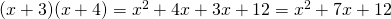We notice thatgives us the; and thatgives us the constant.

To factor a trinomial of the form, we need two valuessuch thatand.

## Example:The factor pairs of 54 are (1,54); (2,27); (3,18); (6,9). Now the last pair has a difference of 3, which is what we need for the middle term.

Letandbecause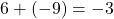and.

Then,# Factor, where## Example 1:

First, let’s examine the expansion of a factored trinomial of this form:.

This time, notice thatas expected, but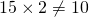. Rather,.

Instead of looking for two numbers that add to 17 and multiply to 10, we need to look for two numbers that add to 17 and multiply to 30.

## Example 2:

Factor:Look for two numbers,and, such that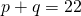and.

The factor pairs of 40 are: (1,40); (2,20); (4,10); (5, 8)

To make 22, we need to use the pair (2,20).

We write a new line of work:(It doesn’t matter if you write thefirst or thefirst).

Now we look for a common factor in the first two terms:Perhaps suprisingly, (explained here), let’s look at the last two terms;Ha!is a common factor! Put together we have:Taking outas a common factor, we have:## Example 3:Find two numbers,andsuch thatand.

Factor pairs of 24 are (1,24); (2,12); (3,8); (4,6). Nowso let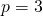and.

Decomposing the middle term we have:Grouping we have:Completing we have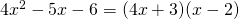.

## Example 4:First, we notice that all three terms share a common factor 3. Let’s factor this out: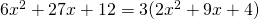Now we factor the reduced trinomial; however theis kept present throughout. You might also try factoring without reducing to see how the result compares.

Now we look forandsuch that, and.

Factor pairs of 8 are (1, 8); (2, 4). To also satisfy, we need to useand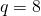.

Decomposing we haveGrouping givesCompleting we have# Try it out:

Correct fields show as green, incorrect as red.

Geogebra link to the old applet

Geogebra link to this applet, for full screen capability

Proof of the method

Done here! Go back to the Factors and Products Menu.